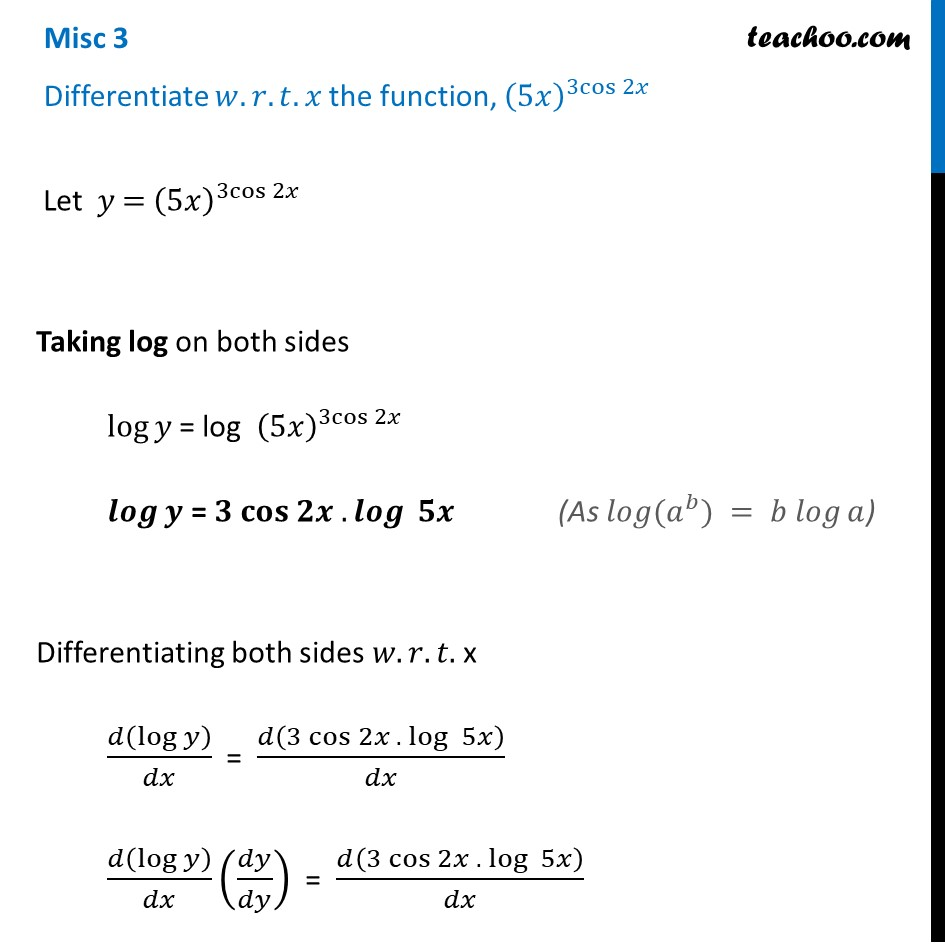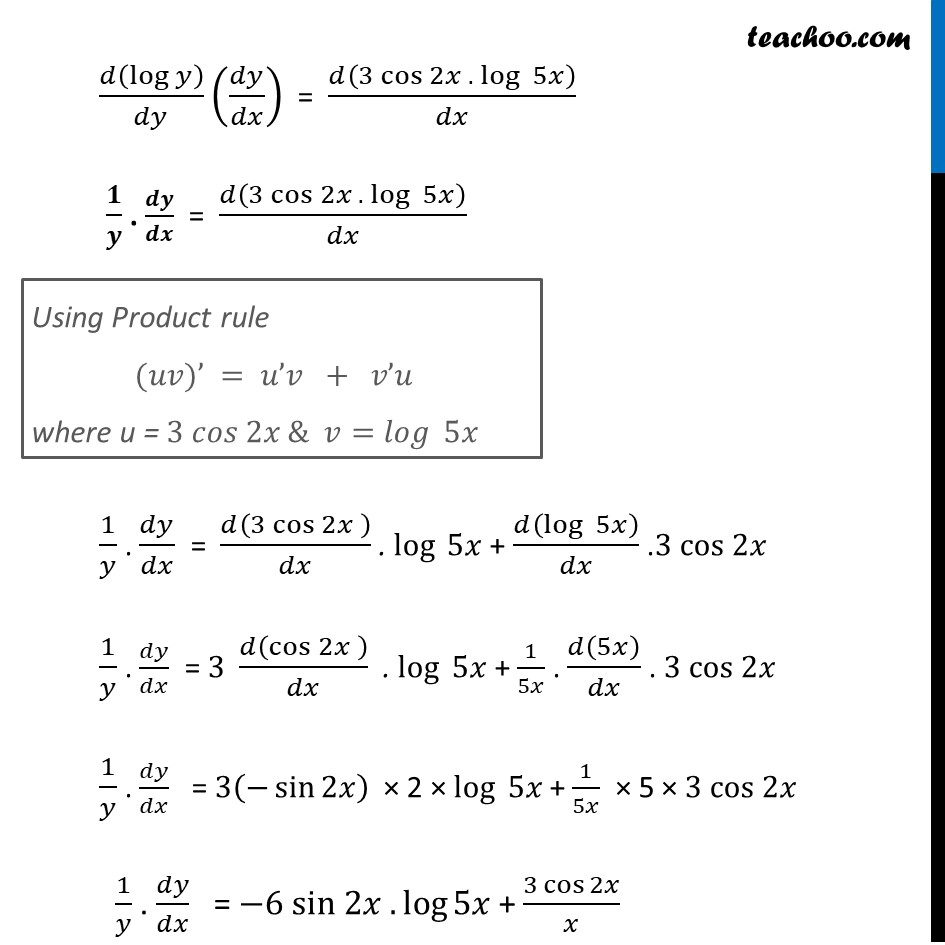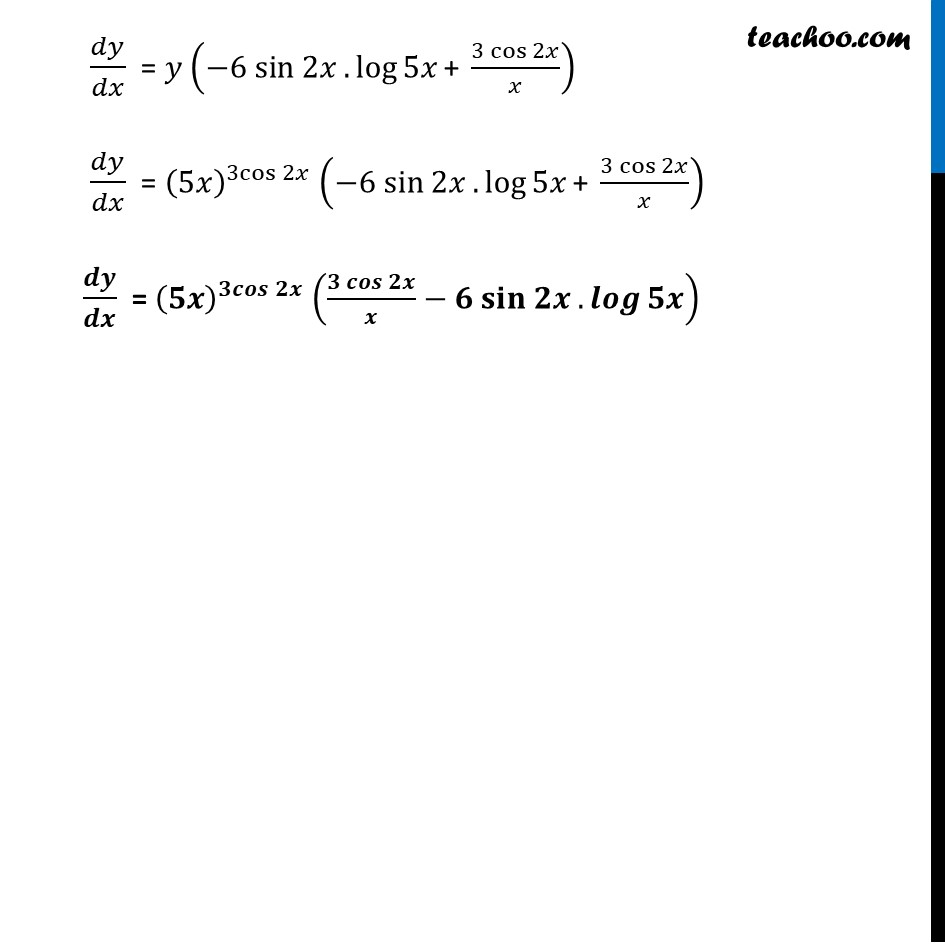Logarithmic Differentiation - Type 1

Chapter 5 Class 12 Continuity and Differentiability
Concept wiseLearn in your speed, with individual attention - Teachoo Maths 1-on-1 Class

### Transcript

Misc 3 Differentiate 𝑤.𝑟.𝑡. 𝑥 the function, (5𝑥)^(3cos⁡2𝑥) Let 𝑦" = " (5𝑥)^(3cos⁡2𝑥) Taking log on both sides log⁡𝑦 = log (5𝑥)^(3cos⁡2𝑥) 𝒍𝒐𝒈⁡𝒚 = 𝟑 𝐜𝐨𝐬 𝟐𝒙 . 𝒍𝒐𝒈 ⁡𝟓𝒙 Differentiating both sides 𝑤.𝑟.𝑡. x 𝑑(log⁡𝑦 )/𝑑𝑥 = 𝑑(3 cos 2𝑥 . log ⁡5𝑥)/𝑑𝑥 𝑑(log⁡𝑦 )/𝑑𝑥 (𝑑𝑦/𝑑𝑦) = 𝑑(3 cos 2𝑥 . log ⁡5𝑥)/𝑑𝑥 (As 𝑙𝑜𝑔⁡(𝑎^𝑏) = 𝑏 𝑙𝑜𝑔⁡𝑎) 𝑑(log⁡𝑦 )/𝑑𝑦 (𝑑𝑦/𝑑𝑥) = 𝑑(3 cos 2𝑥 . log ⁡5𝑥)/𝑑𝑥 𝟏/𝒚 . 𝒅𝒚/𝒅𝒙 = 𝑑(3 cos 2𝑥 . log ⁡5𝑥)/𝑑𝑥 1/𝑦 . 𝑑𝑦/𝑑𝑥 = 𝑑(3 cos 2𝑥 )/𝑑𝑥 . log ⁡5𝑥 + 𝑑(log ⁡5𝑥)/𝑑𝑥 .3 cos 2𝑥 1/𝑦 . 𝑑𝑦/𝑑𝑥 = 3 𝑑(cos 2𝑥 )/𝑑𝑥 . log ⁡5𝑥 + 1/5𝑥 . 𝑑(5𝑥)/𝑑𝑥 . 3 cos 2𝑥 1/𝑦 . 𝑑𝑦/𝑑𝑥 = 3(−sin⁡2𝑥 ) × 2 × log ⁡5𝑥 + 1/5𝑥 × 5 × 3 cos 2𝑥 1/𝑦 . 𝑑𝑦/𝑑𝑥 = −6 sin 2𝑥 . log⁡5𝑥 + (3 cos⁡2𝑥)/𝑥 Using Product rule (𝑢𝑣)’ = 𝑢’𝑣 + 𝑣’𝑢 where u = 3 𝑐𝑜𝑠 2𝑥 & 𝑣=𝑙𝑜𝑔 ⁡5𝑥 𝑑𝑦/𝑑𝑥 = 𝑦 (−6 sin 2𝑥 . log⁡5𝑥 "+ " (3 cos⁡2𝑥)/𝑥) 𝑑𝑦/𝑑𝑥 = (5𝑥)^(3cos⁡2𝑥) (−6 sin 2𝑥 . log⁡5𝑥 "+ " (3 cos⁡2𝑥)/𝑥) 𝒅𝒚/𝒅𝒙 = (𝟓𝒙)^(𝟑𝒄𝒐𝒔⁡𝟐𝒙) ((𝟑 𝒄𝒐𝒔⁡𝟐𝒙)/𝒙−𝟔 𝐬𝐢𝐧 𝟐𝒙 . 𝒍𝒐𝒈⁡𝟓𝒙 )